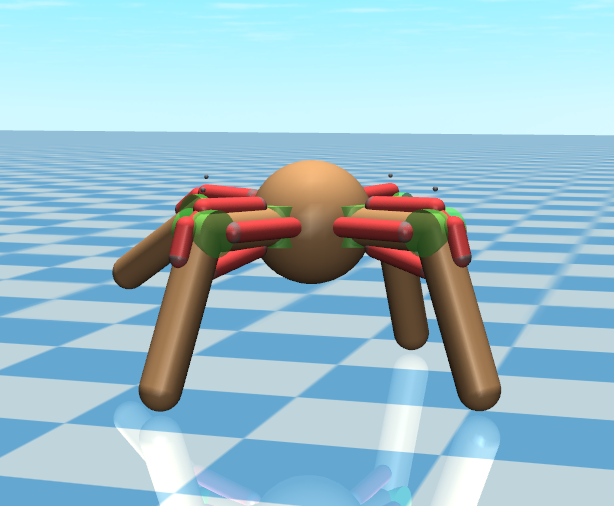Muscle rigged models and environments for machine learning experiments.

# MuscledAgents

OpenAI Gym MuJoCo environments rigged with muscles which can be used with PyMuscle fatigable muscle models.• MuJoCo 1.5
• mujoco-py
• gym

## Setup

python setup.py develop


## Usage

import gym
import muscledagents
import math

def main():

env = gym.make("MuscledAnt-v0")  # Only functional env at the moment
env.reset()

print("Observation Space Dims", env.observation_space.shape)
print("Action Space Dims", env.action_space.shape)

action_size = env.action_space.shape

action = [0.0] * action_size
total_steps = 60 * 10
for i in range(total_steps):
action = ((math.sin(i / 25) + 1) / 2)
action = ((math.sin(i / 35) + 1) / 2)
action = ((math.sin(i / 45) + 1) / 2)
action = ((math.sin(i / 55) + 1) / 2)
env.step(action)
env.render()

if __name__ == '__main__':
main()


To explore these environments and models further you will need to understand how input values get translated into final simulated movements.

### Control Signals

The action space for an ant is continuous control over 16 muscles. For each of four legs there are four muscles. One leg extensor, one leg flexor, and two hip muscles which move the leg left and right (or forward and back depending on your perspective.)

### Gym Environment

The step method takes an array of 16 values which represent the input to the fatigable muscle model for each muscle. Inputs should be in the range [0-1].

### PyMuscle Fatigue

After use muscles produce less force for the same level of input. So if you were to send an input signal which recruited all motor units in a muscle constantly for several seconds the output the model will return will rapidly decrease. A period of light or no use is required for the muscle to recover.

### MuJoCo Model

Each tendon actuator is control limited to the range [-1.0, 0.0]. When a General actuator is tied to a Tendon in MuJoCo negative values are the equivalent of contractions. Muscles cannot produce force in extension so no positive non-zero values are allowed.

Actuators have a gainprm which scales this input value. This is tuned to a value of 100 to work with the mass of the ant and the resistances of opposing tendons.

## Altering the Models

Due to frustration editing XML files the ant model is generated by a python script that uses the mjcf library which I wrote to wrap MuJoCo xml elements in python classes. Note: This may be replaced with the mjcf library from dm_control in the future.

You can view and modify that script in scripts/gen_ant.py.

## Project details

This version0.0.2

Uploaded source
Uploaded py2 py3